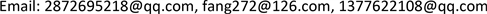﻿ 区间互反判断矩阵权重确定的一种新方法 A Method of Obtaining a Priority Vector from Interval Multiplicative Reciprocal Matrices

Vol.06 No.07(2017), Article ID:22374,8 pages
10.12677/AAM.2017.67098

A Method of Obtaining a Priority Vector from Interval Multiplicative Reciprocal Matrices

Zulin Liu, Fang Liu, Yuhao Wu

School of Mathematics and Information Science, Guangxi University, Nanning GuangxiReceived: Sep. 30th, 2017; accepted: Oct. 14th, 2017; published: Oct. 19th, 2017ABSTRACT

A new method of obtaining a priority vector from interval multiplicative reciprocal matrices is proposed in the present paper. By considering the randomness experienced by decision makers in pairwise comparisons, the cosine maximization method is extended to interval multiplicative reciprocal matrices with approximation-consistency. A new algorithm is proposed to solve the decision making problem with the interval-valued judgements. Numerical results are reported to show the application of the proposed method.

Keywords:Interval Multiplicative Reciprocal Matrix, Priority Vector, Cosine Maximization Method, Approximation-Consistency1. 引言

2. 预备知识

$X=\left\{{x}_{1},{x}_{2},\cdots ,{x}_{n}\right\}$ 表示一组决策方案，经典层次分析法中  ，决策者通过互反判断矩阵表示两两比较的偏好矩阵 $A={\left({a}_{ij}\right)}_{n×n}\subset X×X$ ，其中元素 ${a}_{ij}$ 表示任意方案 ${x}_{i}$${x}_{j}$ 的比值，且 ${a}_{ij}\in \left[1/9,9\right]$ 。当 ${a}_{ij}=9$ 时，表示方案 ${x}_{i}$ 完全优于方案 ${x}_{j}$ ；当 ${a}_{ij}=1/9$ 时，表示方案 ${x}_{j}$ 完全优于方案 ${x}_{i}$ ；当 ${a}_{ij}=1$ 时，表示方案 ${x}_{i}$${x}_{j}$ 无差异。相对测度下，有 ${a}_{ij}\cdot {a}_{ji}=\text{1}$ ，且 ${a}_{ii}=1$ 时， $A={\left({a}_{ij}\right)}_{n×n}$ 称作互反判断矩阵，其一致性定义如下：

$CI=\frac{{\lambda }_{\mathrm{max}}-n}{n-1}$ , $CR=\frac{CI}{RI}$ (1)

$CCI=\frac{{C}_{\mathrm{max}}}{n}$ , ${C}_{\mathrm{max}}=\sum _{j=1}^{n}{C}_{j}=\sum _{j=1}^{n}\sum _{i=1}^{n}\left({\omega }_{i}{a}_{ij}\right)/\left(\sqrt{\sum _{k=1}^{n}{\omega }_{k}^{2}}\sqrt{\sum _{k=1}^{n}{a}_{kj}^{2}}\right)$ (2)

${\stackrel{˜}{A}}^{\sigma }={\left({\stackrel{˜}{a}}_{ij}^{\sigma }\right)}_{n×n}\text{=}\left[\begin{array}{ccccc}& {x}_{\sigma \left(1\right)}& {x}_{\sigma \left(2\right)}& \cdots & {x}_{\sigma \left(n\right)}\\ {x}_{\sigma \left(1\right)}& \left[1,1\right]& \left[{a}_{\sigma \left(1\right)\sigma \left(2\right)}^{-},{a}_{\sigma \left(1\right)\sigma \left(2\right)}^{+}\right]& \cdots & \left[{a}_{\sigma \left(1\right)\sigma \left(n\right)}^{-},{a}_{\sigma \left(1\right)\sigma \left(n\right)}^{+}\right]\\ {x}_{\sigma \left(2\right)}& \left[{a}_{\sigma \left(2\right)\sigma \left(1\right)}^{-},{a}_{\sigma \left(2\right)\sigma \left(1\right)}^{+}\right]& \left[1,1\right]& \cdots & \left[{a}_{\sigma \left(2\right)\sigma \left(n\right)}^{-},{a}_{\sigma \left(2\right)\sigma \left(n\right)}^{+}\right]\\ ⋮& ⋮& ⋮& ⋮& ⋮\\ {x}_{\sigma \left(n\right)}& \left[{a}_{\sigma \left(n\right)\sigma \left(1\right)}^{-},{a}_{\sigma \left(n\right)\sigma \left(1\right)}^{+}\right]& \left[{a}_{\sigma \left(n\right)\sigma \left(2\right)}^{-},{a}_{\sigma \left(n\right)\sigma \left(2\right)}^{+}\right]& \cdots & \left[1,1\right]\end{array}\right]$

${c}_{ij}^{\sigma }=\left\{\begin{array}{l}{a}_{\sigma \left(i\right)\sigma \left(j\right)}^{+},\text{\hspace{0.17em}}\text{\hspace{0.17em}}\text{\hspace{0.17em}}ij\end{array}$ , ${d}_{ij}^{\sigma }=\left\{\begin{array}{l}{a}_{\sigma \left(i\right)\sigma \left(j\right)}^{-},\text{\hspace{0.17em}}\text{\hspace{0.17em}}\text{\hspace{0.17em}}ij\end{array}$ (3)

${E}^{\sigma }\left(\alpha \right)={\left({e}_{ij}^{\sigma }\left(\alpha \right)\right)}_{n×n}={\left({\left({c}_{ij}^{\sigma }\right)}^{\alpha }{\left({d}_{ij}^{\sigma }\right)}^{1-\alpha }\right)}_{n×n}$ (4)

3. 区间数权重确定新方法

${\stackrel{˜}{c}}_{i}^{\sigma }=\sum _{j=1}^{n}\frac{{c}_{ij}^{\sigma }}{\sqrt{\sum _{k=1}^{n}{\left({c}_{kj}^{\sigma }\right)}^{2}}}$ , ${\stackrel{˜}{d}}_{i}^{\sigma }=\sum _{j=1}^{n}\frac{{d}_{ij}^{\sigma }}{\sqrt{\sum _{k=1}^{n}{\left({d}_{kj}^{\sigma }\right)}^{2}}}$ (5)

${\omega }_{i}^{\sigma }\left(C\right)=\frac{{\stackrel{˜}{c}}_{i}^{\sigma }}{\sqrt{\sum _{k=1}^{n}{\left({\stackrel{˜}{c}}_{k}^{\sigma }\right)}^{2}}}/\sum _{i=1}^{n}\frac{{\stackrel{˜}{c}}_{i}^{\sigma }}{\sqrt{\sum _{k=1}^{n}{\left({\stackrel{˜}{c}}_{k}^{\sigma }\right)}^{2}}}$ (6)

${\omega }_{i}^{\sigma }\left(D\right)=\frac{{\stackrel{˜}{d}}_{i}^{\sigma }}{\sqrt{\sum _{k=1}^{n}{\left({\stackrel{˜}{d}}_{k}^{\sigma }\right)}^{2}}}/\sum _{i=1}^{n}\frac{{\stackrel{˜}{d}}_{i}^{\sigma }}{\sqrt{\sum _{k=1}^{n}{\left({\stackrel{˜}{d}}_{k}^{\sigma }\right)}^{2}}}$ (7)

${\stackrel{˜}{t}}_{i}^{\sigma }=\sum _{j=1}^{n}\frac{{\left({c}_{ij}^{\sigma }\right)}^{\alpha }{\left({d}_{ij}^{\sigma }\right)}^{1-\alpha }}{\sqrt{\sum _{k=1}^{n}{\left({\left({c}_{ij}^{\sigma }\right)}^{\alpha }{\left({d}_{ij}^{\sigma }\right)}^{1-\alpha }\right)}^{2}}}$ (8)

${\omega }_{i}^{\sigma }\left(E\right)=\frac{{\stackrel{˜}{t}}_{i}^{\sigma }}{\sqrt{\sum _{k=1}^{n}\left({\stackrel{˜}{t}}_{k}^{\sigma }\right)}}/\sum _{i=1}^{n}\frac{{\stackrel{˜}{t}}_{i}^{\sigma }}{\sqrt{\sum _{k=1}^{n}{\left({\stackrel{˜}{t}}_{k}^{\sigma }\right)}^{2}}}$ (9)

$\alpha =1$ 时，则 ${\omega }_{i}^{\sigma }\left(E\right)={\omega }_{i}^{\sigma }\left(C\right)$ ；当 $\alpha =0$ 时，则 ${\omega }_{i}^{\sigma }\left(E\right)={\omega }_{i}^{\sigma }\left(D\right)$ 。当 $\alpha \in \left(0,1\right)$ 时，对于区间数互反判断矩阵 ${\stackrel{˜}{A}}^{\sigma }$ 中任意一个排列，那么

$\mathrm{min}\left\{{\omega }_{i}^{\sigma }\left(C\right),{\omega }_{i}^{\sigma }\left(D\right)\right\}<{\omega }_{i}^{\sigma }\left(E\right)<\mathrm{max}\left\{{\omega }_{i}^{\sigma }\left(C\right),{\omega }_{i}^{\sigma }\left(D\right)\right\}$ (10)

${\omega }_{i}^{\sigma }=\left[\mathrm{min}\left\{{\omega }_{i}^{\sigma }\left(C\right),{\omega }_{i}^{\sigma }\left(D\right)\right\},\mathrm{max}\left\{{\omega }_{i}^{\sigma }\left(C\right),{\omega }_{i}^{\sigma }\left(D\right)\right\}\right]$ (11)

$\omega =\frac{1}{n!}\sum _{\sigma }\left({\omega }_{1}^{\sigma },{\omega }_{2}^{\sigma },\cdots ,{\omega }_{n}^{\sigma }\right)$ (12)

${\omega }_{j}^{\ast }=\left[\mathrm{min}\left\{1/\sum _{i=1}^{n}{c}_{ij}^{\sigma },1/\sum _{i=1}^{n}{d}_{ij}^{\sigma }\right\},\mathrm{max}\left\{1/\sum _{i=1}^{n}{c}_{ij}^{\sigma },1/\sum _{i=1}^{n}{d}_{ij}^{\sigma }\right\}\right]$ . (13)

${\omega }_{j}^{\sigma }=1/\sum _{i=1}^{n}{e}_{ij}^{\sigma }$ , ${C}_{\mathrm{max}}=n$ , $\forall i,j=1,2,\cdots ,n$ , $\forall \alpha \in \left[0,1\right]$ .

${\omega }_{j}^{\sigma }=1/\sum _{i=1}^{n}{\left({c}_{ij}^{\sigma }\right)}^{\alpha }{\left({d}_{ij}^{\sigma }\right)}^{1-\alpha }$ .

$\alpha =1$ 时，则

${\omega }_{j}^{\sigma }=1/\sum _{i=1}^{n}e{}_{ij}{}^{\sigma }=1/\sum _{i=1}^{n}{c}_{ij}^{\sigma }$ ,

$\alpha =0$ 时，有

${\omega }_{j}^{\sigma }=1/\sum _{i=1}^{n}{e}_{ij}^{\sigma }=1/\sum _{i=1}^{n}{d}_{ij}^{\sigma }$ .

$0<\alpha <1$ 时，则

$\mathrm{min}\left\{\sum _{i=1}^{n}{c}_{ij}^{\sigma },\sum _{i=1}^{n}{d}_{ij}^{\sigma }\right\}<\frac{1}{{\omega }_{j}^{\sigma }}<\mathrm{max}\left\{\sum _{i=1}^{n}{c}_{ij}^{\sigma },\sum _{i=1}^{n}{d}_{ij}^{\sigma }\right\}$

$\mathrm{min}\left\{1/\sum _{i=1}^{n}{c}_{ij}^{\sigma }\text{,}1/\sum _{i=1}^{n}{d}_{ij}^{\sigma }\right\}<{\omega }_{j}^{\sigma }<\mathrm{max}\left\{1/\sum _{i=1}^{n}{c}_{ij}^{\sigma }\text{,}1/\sum _{i=1}^{n}{d}_{ij}^{\sigma }\right\}$

4. 算法与数例

Step 1：假设 $X=\left\{{x}_{1},{x}_{2},\cdots ,{x}_{n}\right\}$ 表示一组有限方案，由方案两两比值构成相应的区间数互反判断矩阵 ${\stackrel{˜}{A}}^{\sigma }={\left({a}_{ij}^{\sigma }\right)}_{n×n}$ ，并利用(3)式从 ${\stackrel{˜}{A}}^{\sigma }$ 中构造两个实互反矩阵 ${C}_{\sigma }$${D}_{\sigma }$

Step 2：利用(2)式检验 ${C}_{\sigma }$${D}_{\sigma }$ ，若存在一个排列 $\sigma$ 使得两者是满意一致的，则直接执行Step 6，否则继续执行下一步。

Step 3：首先利用(3)式获得 ${C}_{\sigma }$${D}_{\sigma }$ 的权重向量 ${\omega }_{\sigma }=\left({\omega }_{1}^{\sigma },{\omega }_{2}^{\sigma },\cdots ,{\omega }_{n}^{\sigma }\right)$ 。然后利用欧几里得距离获得 ${C}_{\sigma }$${D}_{\sigma }$ 中的元素与其对应权重的平均偏差值：

$\overline{X}=\frac{{\left[\sum _{i=1}^{n}\sum _{j=1}^{n}{\left({e}_{ij}^{\sigma }-{\omega }_{i}/{\omega }_{j}\right)}^{2}\right]}^{1/2}}{{n}^{2}}$

Step 4：令 ${\overline{b}}_{kl}=\mathrm{max}\left\{{\overline{c}}_{ij}^{\sigma }\right\}$ ，那么

${c}_{ij}^{\sigma }=\left\{\begin{array}{l}\mu \cdot {c}_{kl}^{\sigma }+\left(1-\mu \right)\cdot \left(\frac{{\omega }_{k}^{\sigma }}{{\omega }_{l}^{\sigma }}\right),\text{\hspace{0.17em}}\text{\hspace{0.17em}}\text{\hspace{0.17em}}k=i,l=j\\ {c}_{ij}^{\sigma }\text{\hspace{0.17em}}\text{\hspace{0.17em}}\text{\hspace{0.17em}}\text{\hspace{0.17em}}\text{\hspace{0.17em}}\text{\hspace{0.17em}}\text{\hspace{0.17em}}\text{\hspace{0.17em}}\text{\hspace{0.17em}}\text{\hspace{0.17em}}\text{\hspace{0.17em}}\text{\hspace{0.17em}}\text{\hspace{0.17em}}\text{\hspace{0.17em}}\text{\hspace{0.17em}}\text{\hspace{0.17em}}\text{\hspace{0.17em}}\text{\hspace{0.17em}}\text{\hspace{0.17em}}\text{\hspace{0.17em}}\text{\hspace{0.17em}}\text{\hspace{0.17em}}\text{\hspace{0.17em}}\text{\hspace{0.17em}}\text{\hspace{0.17em}}\text{\hspace{0.17em}}\text{\hspace{0.17em}}\text{\hspace{0.17em}}\text{\hspace{0.17em}}\text{\hspace{0.17em}}\text{\hspace{0.17em}}\text{otherwise}\end{array}$

Step 5：改变下标 $k$$l$ 的值，返回Step 2。

Step 6：应用(12)式获得区间数互反判断矩阵 ${\stackrel{˜}{A}}^{\sigma }={\left({a}_{ij}^{\sigma }\right)}_{n×n}$ 权重向量。

Step 7：利用文献  的可能度公式确定相应的可能度矩阵 $P$

Step 8：利用文献  中的行列消去法可获得方案权重的排序。

Step 9：结束。

${\stackrel{˜}{A}}_{2}^{\sigma }=\left[\begin{array}{cccc}\left[1,1\right]& \left[2,3\right]& \left[4,9\right]& \left[1,2\right]\\ \left[1/3\right]& \left[1,1\right]& \left[1,2\right]& \left[3,8\right]\\ \left[1/9,1/4\right]& \left[1/2,1\right]& \left[1,1\right]& \left[2,5\right]\\ \left[1/2,1\right]& \left[1/8,1/3\right]& \left[1/5,1/2\right]& \left[1,1\right]\end{array}\right]$

${C}_{{\sigma }_{3}}=\left[\begin{array}{cccc}1& 1& 2& 4\\ 1& 1& 1/8& 1/5\\ 1/2& 8& 1& 1\\ 1/4& 5& 1& 1\end{array}\right]$ , ${D}_{{\sigma }_{3}}=\left[\begin{array}{cccc}1& 2& 3& 9\\ 1/2& 1& 1/3& 1/2\\ 1/3& 3& 1& 2\\ 1/9& 2& 1/2& 1\end{array}\right]$

$\overline{E}=\left[\begin{array}{cccc}0& 1.9287& 0.6084& 2.1082\\ 0.6586& 0& 0.3501& 0.4459\\ 0.2186& 5.8954& 0& 0.3595\\ 0.2786& 3.4519& 0.2644& 0\end{array}\right]$

${{C}^{\prime }}_{{\sigma }_{3}}=\left[\begin{array}{cccc}1& 1& 2& 4\\ 1& 1& 0.2785& 0.2\\ 0.5& 3.5904& 1& 1\\ 0.25& 5& 1& 1\end{array}\right]$${\omega }_{\sigma }=\left(\left[0.3181,0.4631\right],\left[0.236,0.2984\right],\left[0.134,0.1902\right],\left[0.1075,0.2109\right]\right)$

$P=\left[\begin{array}{cccc}0.5& 1& 1& 1\\ 0& 0.5& 1& 1\\ 0& 0& 0.5& 0.528\\ 0& 0& 0.472& 0.5\end{array}\right]$

5. 结论

A Method of Obtaining a Priority Vector from Interval Multiplicative Reciprocal Matrices[J]. 应用数学进展, 2017, 06(07): 808-815. http://dx.doi.org/10.12677/AAM.2017.67098

1. 1. Saaty, T.L. and Vargas, L.G. (1987) Uncertainty and Rank Order in the Analytic Hierarchy Process. European Journal of Operational Research, 32, 107-117.

2. 2. Wang, Y.M., Yang, J.B. and Xu, D.L. (2005) A Two-Stage Logarithmic Goal Programming Method for Generating Weights from Interval Comparison Matrices. Fuzzy Sets and Systems, 152, 475-498.

3. 3. Liu, F. (2009) Acceptable Consistency Analysis of Interval Reciprocal Comparison Matrices. Fuzzy Sets and Systems, 160, 2686-2700.

4. 4. Xia, M.M. and Chen, J. (2015) Studies on Interval Multiplicative Preference Relations and Their Application to Group Decision Making. Group Decision and Negotiation, 24, 115-144. https://doi.org/10.1007/s10726-014-9383-9

5. 5. Saaty, T.L. (1980) The Analytic Hierarchy Process. McGraw-Hill, New York.

6. 6. Xu, Z.S. and Wei, C.P. (1999) A Consistency Improving Method in the Analytic Hierarchy Process. European Journal of Operational Research, 116, 443-449.

7. 7. Liu, F., Pedrycz, W. and Zhang, W.G. (2017) Limited Ra-tionality and Its Quantification through the Interval Number Judgments with Permutations. IEEE Transactions on Cybernetics. (In Press)

8. 8. Meng, F, Chen, X., Zhu, M. and Lin, J. (2015) Two New Methods for Deriving the Priority Vector from Interval Mul-tiplicative Preference Relations. Information Fusion, 26, 122-135.

9. 9. Kou, G. and Lin, C. (2014) A Cosine Maximization Method for the Priority Vector Derivation in AHP. European Journal of Operational Research, 235, 225-232.